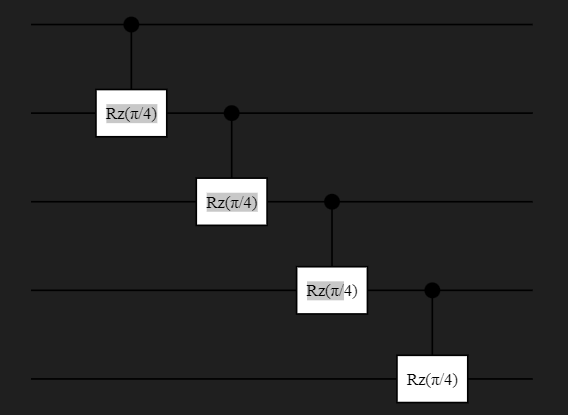# How to create a single shared parameter for multiple gates within a circuit directly using Yao.jl?

I am trying to parameterize a circuit using a single variable `θ` in the code below but the output is:
` Number of parameters in the circuit: 4`.

Can someone please help me create a single shared parameter for multiple gates within a circuit directly?

``````using Yao
using YaoPlots

# Define the number of qubits
n = 5

# Define a single parameter for all Rz gates
θ = π/4  # replace this with your desired angle

# Create a chain block to hold our circuit
circuit = chain(n)

# Apply controlled Rz gates with the shared parameter gate
for i in 1:n-1
push!(circuit, control(i, i+1 => Rz(θ)))
end

# Check the number of parameters in the circuit
println("Number of parameters in the circuit: ", length(parameters(circuit)))
plot(circuit)
``````

Hi, this is one limitation of Yao currently, you need to do either the following

• sum up the gradient yourself (recommend in simple cases)
• use a general-purpose AD engine e.g Zygote

there is an example in our test for Zygote if you want to do the second approach

1 Like

For training with shared parameters, Yao assume the parameter sharing is automatically handled by your AD engine such as Zygote. Here is an example: https://github.com/QuantumBFS/QuAlgorithmZoo.jl/blob/master/examples/PortZygote/shared_parameters.jl

If you want a real parameter sharing, I would suggest using symbol engine:

``````julia> using Yao, SymEngine

julia> @vars θ
(θ,)

julia> # Create a chain block to hold our circuit
circuit = chain(n)

# Apply controlled Rz gates with the shared parameter gate
nqubits: 5
chain

julia> for i in 1:n-1
push!(circuit, control(i, i+1 => Rz(θ)))
end

julia> circuit
nqubits: 5
chain
├─ control(1)
│  └─ (2,) rot(Z, θ)
├─ control(2)
│  └─ (3,) rot(Z, θ)
├─ control(3)
│  └─ (4,) rot(Z, θ)
└─ control(4)
└─ (5,) rot(Z, θ)

julia> subs(circuit, θ=>0.4)
nqubits: 5
chain
├─ control(1)
│  └─ (2,) rot(Z, 0.4)
├─ control(2)
│  └─ (3,) rot(Z, 0.4)
├─ control(3)
│  └─ (4,) rot(Z, 0.4)
└─ control(4)
└─ (5,) rot(Z, 0.4)
``````

Dear @Roger-luo and @1115 thank you for your response.